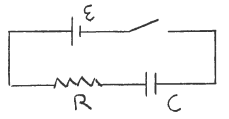# Problem: In the circuit show in the sketch the battery emf is ε = 48.0 V, R = 8.0 Ω, and C = 4.0 x 10-6 F. Initially the switch is open and the charge on the capacitor is zero. At time l after the switch is closed, the current in the resistor is 2.00 A. When the current has this value, what is the charge q on the capacitor? A) zero B) 64.0 x 10-6 C C) 128 x 10-6 C D) 192 x 10-6 C E) None of the above answers

###### FREE Expert Solution
98% (298 ratings)
###### Problem Details

In the circuit show in the sketch the battery emf is ε = 48.0 V, R = 8.0 Ω, and C = 4.0 x 10-6 F. Initially the switch is open and the charge on the capacitor is zero. At time l after the switch is closed, the current in the resistor is 2.00 A. When the current has this value, what is the charge q on the capacitor?

A) zero

B) 64.0 x 10-6 C

C) 128 x 10-6 C

D) 192 x 10-6 C

E) None of the above answersFrequently Asked Questions

What scientific concept do you need to know in order to solve this problem?

Our tutors have indicated that to solve this problem you will need to apply the !! Resistor-Capacitor Circuits concept. If you need more !! Resistor-Capacitor Circuits practice, you can also practice !! Resistor-Capacitor Circuits practice problems.

How long does this problem take to solve?

Our expert Physics tutor, Douglas took 10 minutes and 9 seconds to solve this problem. You can follow their steps in the video explanation above.